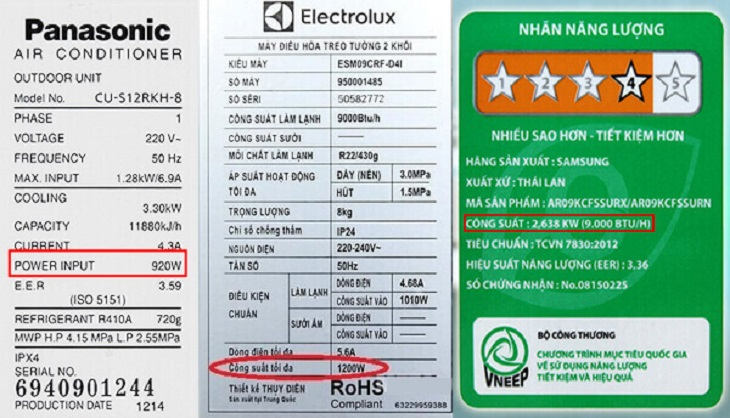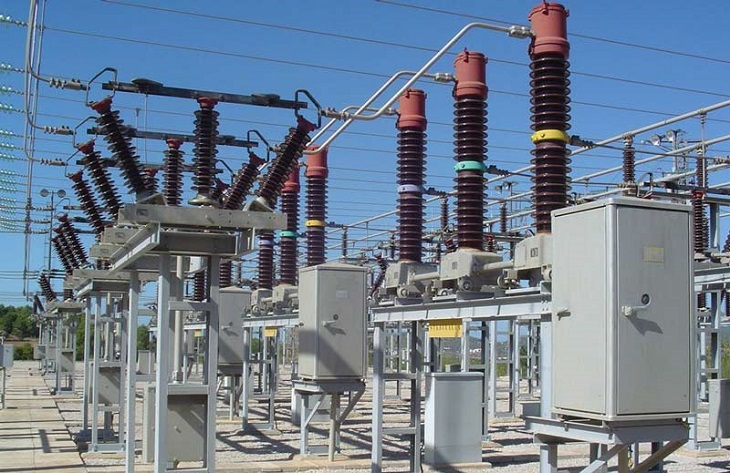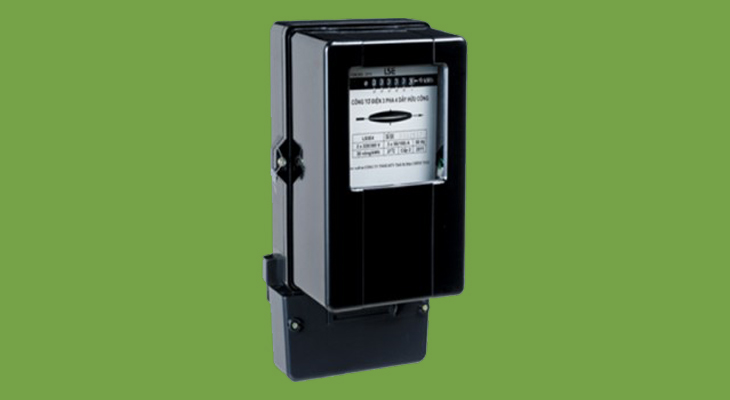# Calculating Electric Consumption Power: A Step-by-Step Guide

Want to lower your electricity bill and find the right devices for your home? Look no further - here is our comprehensive guide on calculating power consumption. Learn all you need to know with us!

0
56

### 1. What is power?

Power, symbolized by P (abbreviation for Potestas), is the measure of work done in a unit of time. It can be classified into mechanical power and consumption power:

• Mechanical power is used in uniform motion and rotational motion.
• Consumption power is the energy of electrical energy transformed into useful energy.In practical terms, power indicates the amount of energy consumed by electrical appliances in a unit of time.

### 2. How to calculate power consumption

Most manufacturers specify the operating power of electrical appliances. This information allows you to easily calculate the amount of electricity consumed in a month, helping you plan your electricity usage according to your family’s budget.

The formula for calculating power consumption is as follows:

A = Amount of electricity consumed (KWh)
P = Consumption power (KW)
t = Time of use (h)

Conversion to W:

• 1KW = 1000W
• 1MW = 1,000,000W

Example:

Let’s say an air conditioner has a power of 120W (0.12KW) and operates for one day (24h). The amount of electricity consumed by the unit would be 0.12 x 24 = 2.88 (KWh).In addition, if the product’s energy label mentions energy consumption, you can also calculate the amount of electricity consumed by the device in one day.

Example:

Let’s say the energy consumption of a refrigerator is 381 KWh/year. The amount of electricity consumed by the refrigerator in one day would be 381 (KWh) / 365 (days) ≈ 1.04 (KWh).

### 3. How to calculate 3-phase power

#### What is 3-phase power?

3-phase power is a high-power and high-energy consumption current. The voltage (V) at each location in the world may vary. In Vietnam, the standard voltage for 3-phase power is 360V.

This type of current is more costly compared to single-phase power and is typically used for industrial machinery such as car washers, industrial sewing machines, and industrial washing machines.#### Formula for calculating 3-phase power

Each type of industrial machine has an inspection label and electrical consumption parameters on the machine body. You can calculate 3-phase power in 2 ways:

Method 1:

A = U x I x t

Method 2:

A = U x I x t x Cosφ